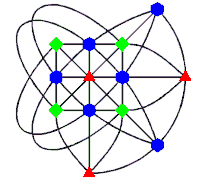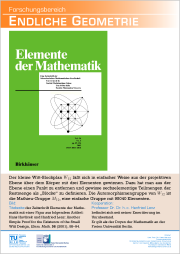# Design Theory

We exhibit finite combinatorial structures, like designs and divisible designs.

## External Partners

Andrea Blunck, Department Mathematik, Universität Hamburg, Germany.

Sabine Giese, Hanfried Lenz, and Ralph-Hardo Schulz, Mathematisches Institut, Freie Universität Berlin, Germany.

Corrado Zanella, Dipartimento di Tecnica e Gestione dei Sistemi Industriali, Università di Padova, Vicenza, Italy.A conic in PG(2,3)

## The Veronese Surface in PG(5,3) and the Small Witt Design

The picture on the left hand side shows the projective plane over GF(3), i.e. the field of residue classes modulo three. This plane has thirteen points (the coloured polygons) and thirteen lines (the black curves). Each point is on exactly four lines and each line carries exactly four points.

The four green squares comprise a conic in that plane. It has four tangent lines (meeting the conic in one point only), three interior lines (missing the conic), and six external lines (meeting the conic in exactly two points). Dually, there are three internal points (lying on no tangent) and six external points (lying on exactly two tangents). Those points are illustrated by red triangles and blue hexagons, respectively. It is a peculiar property, that the green conic and the red triangle determine each other uniquely.

This property is the backbone of some of our results on the relationship between the Veronese surface in the 5-dimensional space over GF(3) and a 12-cap in that space which is a point model for the Witt design W12, a combinatorial structure with twelve points and 132 blocks. As a matter of fact, this 12-cap has been found before by H.S.M. Coxeter using a completely different approach.

## Publications

• H. Havlicek: The Veronese surface in PG(5,3) and Witt's 5-(12,6,1) design, J. Comb. Theor. Ser. A. 84 (1998), 87-94.
Preprint (PDF)
• H. Havlicek: Giuseppe Veronese and Ernst Witt - neighbours in PG(5,3), Aequat. Math. 58 (1999), 85-92.
Preprint (PDF)
• H. Havlicek: Giuseppe Veronese and Ernst Witt - neighbours in PG(5,3), Eighth international conference on geometry, Nahsholim (Israel) 1999, J. Geom. 65 (1999), p. 9. (Abstract)
• H. Havlicek: A model of the Witt design W12 based on quadrics of PG(2,3), Discrete Math. 255 (2002), 189-192.
Preprint (PDF)
• H. Havlicek and H. Lenz: Another simple proof for the existence of the small Witt design, Elem. Math. 56 (2001), 89-94.
Preprint (PDF)
• S. Giese, H. Havlicek and R.-H. Schulz: Some constructions of divisible designs from Laguerre geometries, Discrete Math. 301 (2005), 74-82.
Preprint (PDF)
• H. Havlicek: Divisible Designs, Laguerre Geometry, and Beyond, Summer School on Combinatorial Geometry and Optimisation 2004 "Giuseppe Tallini", Brescia, Italy, July 4th-10th, 2004. Quaderni del Seminario Matematico di Brescia 11 (2006), iv+63 pp. (electronic).
Preprint (PDF)
• A. Blunck, H. Havlicek, and C. Zanella: Lifting of divisible designs, Des. Codes Cryptogr., 42 (2007), 1-14.
Preprint (PDF)
• A. Blunck, H. Havlicek, and C. Zanella: Divisible designs from twisted dual numbers, Des. Codes Cryptogr. 47 (2008), 11-20.
Preprint (PDF)

Andrea Blunck
Hans Havlicek
Chain Geometry
Geometry and Quantum Theory
Veronese Varieties
Sitemap

Ralph-Hardo Schulz, Free University of BerlinEndliche Geometrie